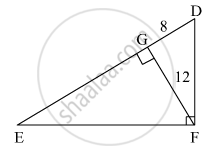Share

# In the Given Figure, ∠Dfe = 90°, Fg ⊥ Ed, If Gd = 8, Fg = 12, Find (1) Eg (2) Fd and (3) Ef - Geometry

ConceptPythagoras Theorem

#### Question

In the given figure, ∠DFE = 90°, FG ⊥ ED, If GD = 8, FG = 12, find (1) EG (2) FD and (3) EF#### Solution

We know that,
In a right angled triangle, the perpendicular segment to the hypotenuse from the opposite vertex, is the geometric mean of the segments into which the hypotenuse is divided.
Here, seg GF ⊥ seg ED

$\therefore {GF}^2 = EG \times GD$
$\Rightarrow {12}^2 = EG \times 8$
$\Rightarrow 144 = EG \times 8$
$\Rightarrow EG = \frac{144}{8}$
$\Rightarrow EG = 18$

Hence, EG = 18.
Now,
According to Pythagoras theorem, in ∆DGF

${DG}^2 + {GF}^2 = {FD}^2$
$\Rightarrow 8^2 + {12}^2 = {FD}^2$
$\Rightarrow 64 + 144 = {FD}^2$
$\Rightarrow {FD}^2 = 208$
$\Rightarrow FD = 4\sqrt{13}$

In ∆EGF

${EG}^2 + {GF}^2 = {EF}^2$
$\Rightarrow {18}^2 + {12}^2 = {EF}^2$
$\Rightarrow 324 + 144 = {EF}^2$
$\Rightarrow {EF}^2 = 468$
$\Rightarrow EF = 6\sqrt{13}$

Hence, FD =$4\sqrt{13}$  and  EF=$6\sqrt{13}$

Is there an error in this question or solution?

#### Video TutorialsVIEW ALL 

Solution In the Given Figure, ∠Dfe = 90°, Fg ⊥ Ed, If Gd = 8, Fg = 12, Find (1) Eg (2) Fd and (3) Ef Concept: Pythagoras Theorem.
S